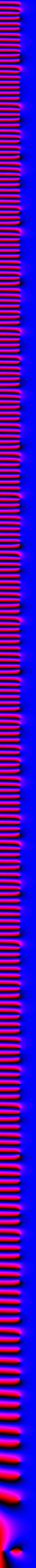## Page 3: The Mandelbrot set iteration

 The iteration used to compute the Mandelbrot set is z := z^2 + c, starting from z = 0. Therefore the first few iterations give c, c^2+c, (c^2+c)^2+c, ... a sequence of polynomials in c that are related to the Mandelbrot set. For this page, the scale has been changed to 60 pixels/unit and the new range goes from -2.5 to 2.5.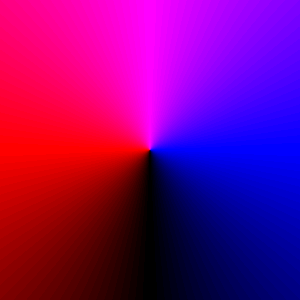f(c) = c. (1 iteration). Degree 1, therefore 1 zero.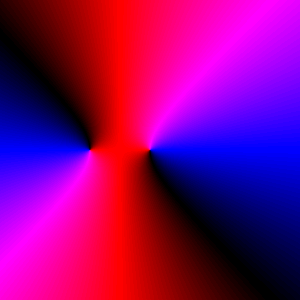f(c) = c^2 + c. (2 iterations). Degree 2.f(c) = (c^2 + c)^2 + c. (3 iterations). Degree 4.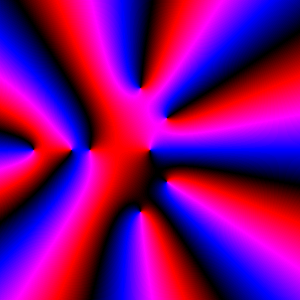f(c) = [(c^2 + c)^2 + c]^2 + c. (4 iterations). Degree 8.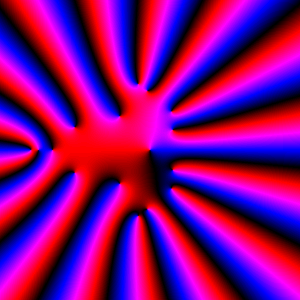(5 iterations). Degree 16.(7 iterations). Degree 64.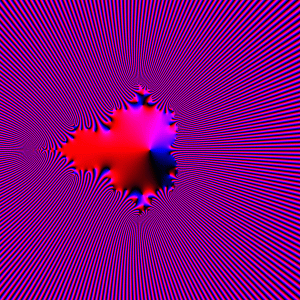(9 iterations). Degree 256.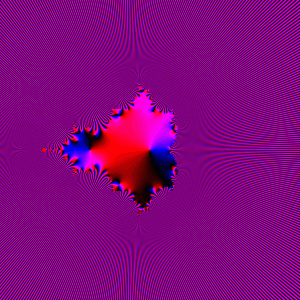(10 iterations). Degree 512. Note that the inside colors do not really converge, for instance the left internal zero is present only when the iteration number is even. Other internal zeros follow other patterns.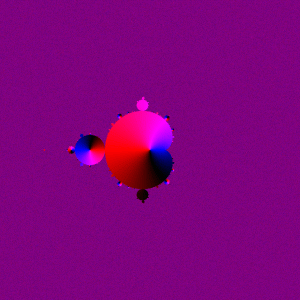(1000 iterations). The grainy purple color is not a specific phase, it is a mix of blue, magenta, red and black.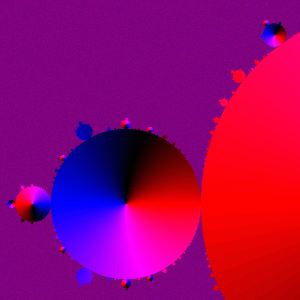A zoom on the previous image. Some disks have a zero at their centers, others have none.

page last updated: March 16, 2002